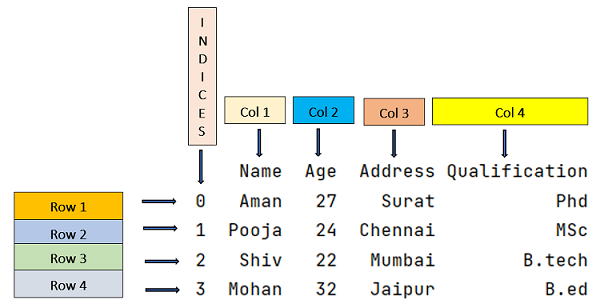# How to select multiple rows from a Pandas DataFrame?

Given a DataFrame, we have to select multiple rows from a Pandas DataFrame. By Pranit Sharma Last updated : April 12, 2023

## Select multiple rows from a Pandas DataFrame

The pandas.DataFrame.loc property allows us to select a row by its column value. To select multiple rows, we can also use the loc[] property by defining the number of rows along with column names (in case we don't need all the columns).

### Syntax

Use the following syntax to select multiple rows:

```DataFrame.loc[x:y,['col-name1',col-name2]]
```

Here, x and y are starting and ending indexes respectively. x represents the first row whereas y represents the last row.

Let us understand with the help of some examples.

## Example 1: Select two rows and two columns

```# Importing pandas package
import pandas as pd

# Creating a dictionary of student marks
d = {
"Peter":[65,70,70,75],
"Harry":[45,56,66,66],
"Tom":[67,87,65,53],
"John":[56,78,65,64]
}

# Now we will create DataFrame, and
df = pd.DataFrame(d,index=["Maths","Physics","Chemistry","English"])

# Printing the DataFrame
print("DataFrame:","\n",df,"\n")

# Printing the selected rows
print("Selected rows:","\n",df.loc[['Maths','Physics'],['Peter',"Harry"]])
```

### Output

```DataFrame:
Peter  Harry  Tom  John
Maths         65     45   67    56
Physics       70     56   87    78
Chemistry     70     66   65    65
English       75     66   53    64

Selected rows:
Peter  Harry
Maths       65     45
Physics     70     56
```

### Explanation

Since we have changed the index names to subject names while creating the DataFrame, we need to pass the list of rows up to which we want to view our data.

## Example 2: Select all the rows and just one column

```# Importing pandas package
import pandas as pd

# Creating a dictionary of student marks
d = {
"Peter":[65,70,70,75],
"Harry":[45,56,66,66],
"Tom":[67,87,65,53],
"John":[56,78,65,64]
}

# Now we will create DataFrame, and
df = pd.DataFrame(d,index=["Maths","Physics","Chemistry","English"])

# Printing the DataFrame
print("DataFrame:","\n",df,"\n")

# Printing marks of peter in all the subjects i.e.,
# selecting all the rows but just one column.
print ("Selected rows:","\n",df.loc[['Maths','Physics','Chemistry','English'],['Peter']])
```

### Output

```DataFrame:
Peter  Harry  Tom  John
Maths         65     45   67    56
Physics       70     56   87    78
Chemistry     70     66   65    65
English       75     66   53    64

Selected rows:
Peter
Maths         65
Physics       70
Chemistry     70
English       75
```

### Explanation

Here, we need to pass a list of all the index names to select all the rows. If we want all the column names to be printed so we do not need to define a specific list of column names.

Note: In case we have a large data set, we do not assign specific index names and hence in this case multiple rows can be selected by passing sliced index in loc[] property.

## Example 3: Select multiple rows by passing sliced index

```# Importing pandas package
import pandas as pd

# Creating a dictionary
d = {
'Name':['Aman', 'Pooja', 'Shiv', 'Mohan'],
'Age':[27, 24, 22, 32],
'Qualification':['Phd', 'MSc', 'B.Tech', 'B.Ed.']
}

# Convert the dictionary into DataFrame
df = pd.DataFrame(d)

# Select three rows and two columns
print(df.loc[1:3, ['Name', 'Qualification']])
```

### Output

```    Name Qualification
1  Pooja           MSc
2   Shiv        B.Tech
3  Mohan         B.Ed.
```

### Explanation

We know that the index ranges from 0 to n-1, where n is the number of rows. In the above example, we will pass a sliced index (1:3) instead of list of index names to select two rows (2nd row and 3rd row) and we will pass a list of specific column names that we want to display.